Courses

# CBSE Class 3 Maths Worksheet- Where To Look From Notes | EduRev

## Class 3 : CBSE Class 3 Maths Worksheet- Where To Look From Notes | EduRev

The document CBSE Class 3 Maths Worksheet- Where To Look From Notes | EduRev is a part of the Class 3 Course Mathematics for Class 3: NCERT.
All you need of Class 3 at this link: Class 3

Q.1. Tell whether the dotted line on each shape represents a line of symmetry. Write yes or no.
(a)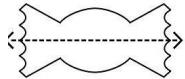(b)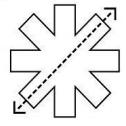(c)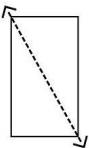(d)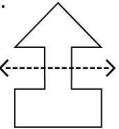Ans.

(a) Yes
(b) Yes
(c) No
(d) No

Q.2. Draw lines of symmetry on the shapes below.  Some shapes may have more than one line of symmetry.
(a)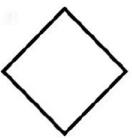(b)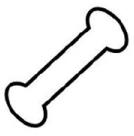(c)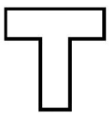(d)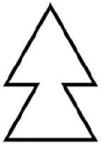(e)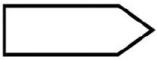(f)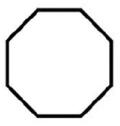(g)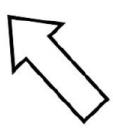(h)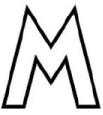(i)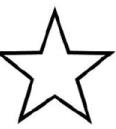Ans.
(a)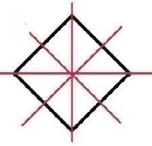(b)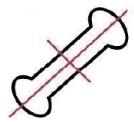(c)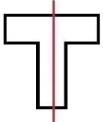(d)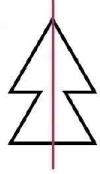(e)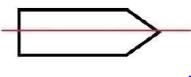(f)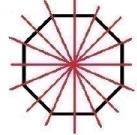(g)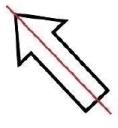(h)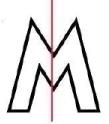(i)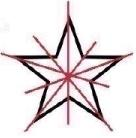Q.3. Draw the missing half of each shape. Each shape should be reflected on its line of symmetry.
1.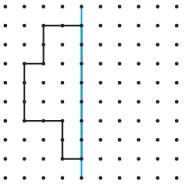2.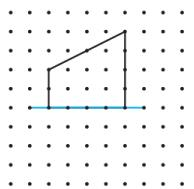3.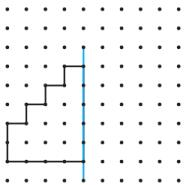4.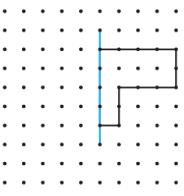5.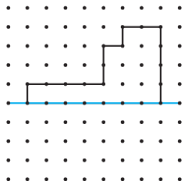6.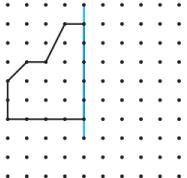Ans.
1.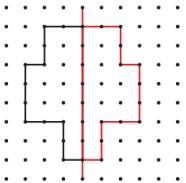2.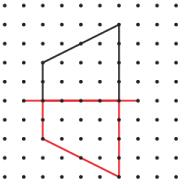3.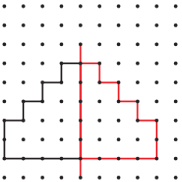4.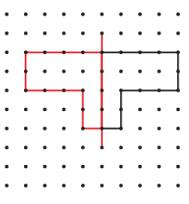5.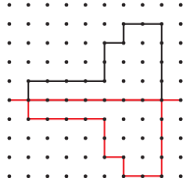6.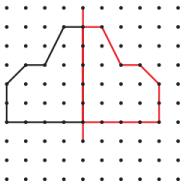Q.4. Tell whether the dotted line on each shape is a line of symmetry.  Write yes or no.
(a)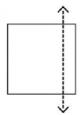(b)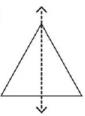(c)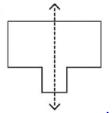(d)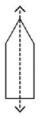(e)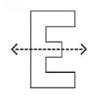(f)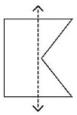(g)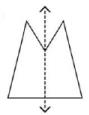(h)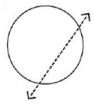(i)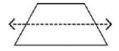(j)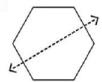(k)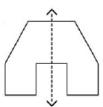(l)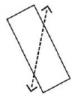Ans.
(a) No
(b) Yes
(c) Yes
(d) Yes
(e) Yes
(f) No
(g) Yes
(h) No
(i) No
(j) Yes
(k) Yes
(l) No

Q.5. Draw a line of symmetry on each shape.
(a)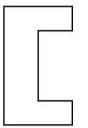(b)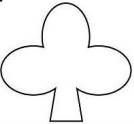(c)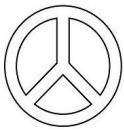(d)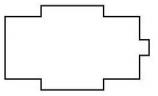Ans.

(a)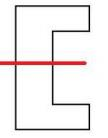(b)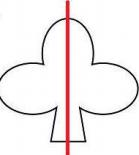(c)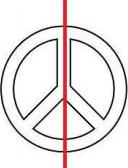(d)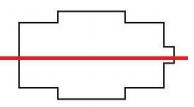Q.6. Draw the second half of each symmetrical shape.
(a)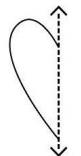(b)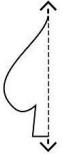(c)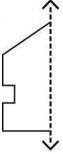(d)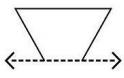Ans.

(a)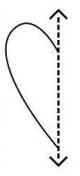(b)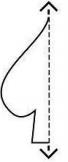(c)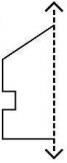(d)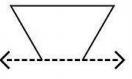Offer running on EduRev: Apply code STAYHOME200 to get INR 200 off on our premium plan EduRev Infinity!

## Mathematics for Class 3: NCERT

56 videos|42 docs|44 tests

,

,

,

,

,

,

,

,

,

,

,

,

,

,

,

,

,

,

,

,

,

;